（本單元範例程式全由李明憲老師撰寫，供各位同學參考）

(1) 運算迭代

exit 指令

IFS 類型的碎形會需要用到亂數產生器， 普遍而言一個亂數產生器每次被呼叫就會給出一個 0 到 1 之間的不同實數，其出現的機率是相當平均的。我們這裏使用 Numerical Recipes 書中建議提供的 ran2，它均勻度的品質是最好的。另有一個 ran3，其速度比 ran2 快很多。（如果你使用的是 Fortran90 或更新的版本，就有內建的亂數函式可用。）

(2) 圖形

paper and graph setting

point symbol type

a. coloring

cyclic index

b. magnification

repeating

(3) Repeating

A simple "GOTO" application

Mandelbrot 集合

pgopen 與 pgclos：開啟及關閉繪圖裝置

papap 與 pgenv：控制圖面的大小以及邊框範圍

pgpt：畫點

pgbbuf 與 pgebuf：開啟及關閉繪圖緩衝區

program mandelbrot_simple
implicit none
complex c,z_ini,z
integer pgopen,i,j,k,isymbol,n_gen
real c_x_min,c_x_max,c_y_min,c_y_max,c_x,c_y,abs_z

isymbol = -1

write (*,*) 'The program plot Mandelbrot set in a simple way.'
write (*,*)
z_ini = (0.0,0.0)

if ( pgopen('/xwin') .le. 0 ) stop
call pgpap(5.0,1.0)

write (*,*) 'What is the numer of iteration for the mapping ?'

c_x_min = -2.0
c_x_max = 0.5
c_y_min = -1.25
c_y_max = 1.25

call pgenv(c_x_min, c_x_max, c_y_min, c_y_max, 1, 0)

do j=1,500
c_x = c_x_min + j*(c_x_max-c_x_min)/500
call pgbbuf
do k=1,500
c_y = c_y_min + k*(c_y_max-c_y_min)/500
c = (1.0,0.0)*c_x + (0.0,1.0)*c_y
z = z_ini

do i=1,n_gen
z = z*z + c
abs_z = conjg(z)*z
if (abs_z.gt.10e+10) then
call pgpt(1,c_x,c_y,isymbol)
exit
endif

end do
end do
call pgebuf
end do

call pgclos
end

mandelbrot.f mandelbrot.x mandelbrot.f.txt

Julia Set

Julia Set 產生的方法和 Mandelblort Set 一樣是檢驗迭代 f(z) = z*z + c 的發散性，但是是掃遍不同的 z 而不是 c。下圖左右分別是 c = (0.2,0.6) 及 c = (0.0,1.0) 的結果：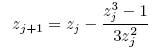z3 - 1 = 0 要求滿足這個方程式的解，一定是具有 2π/3 對稱性的。

newton.f newton.x newton.f.txt

Koch 曲線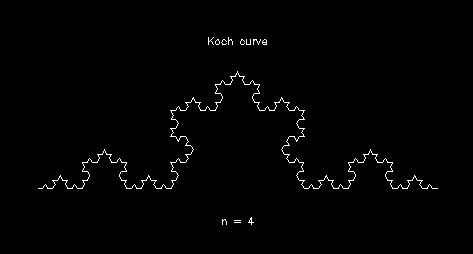koch_snow.f koch_snow.f.txt koch_snow.x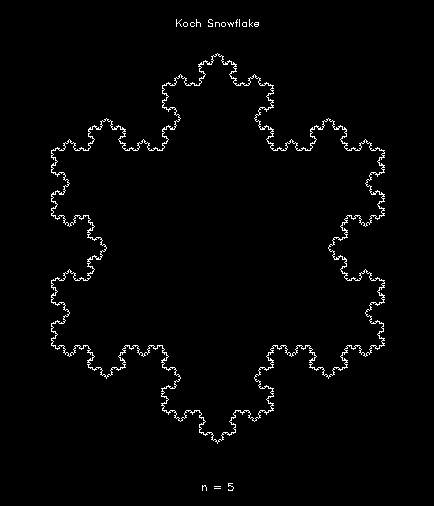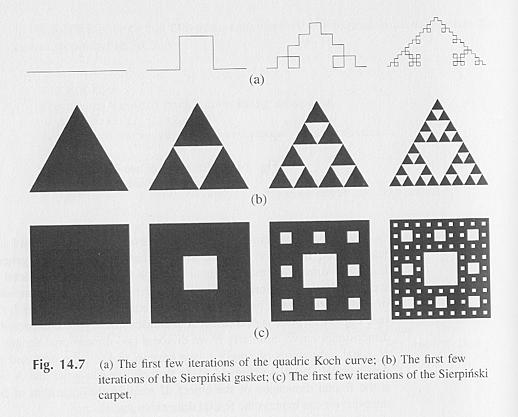xn+1 = axn + byn + e

yn+1 = cxn + dyn + f

 a b c d e f p 0.00 0.00 0.00 0.16 0.00 0.00 0.01 0.85 0.04 -0.04 0.85 0.00 1.60 0.85 0.20 -0.26 0.23 0.22 0.00 1.60 0.07 -0.15 0.28 0.26 0.24 0.00 0.44 0.07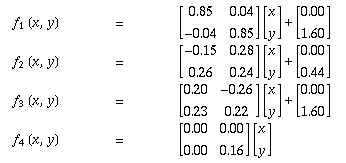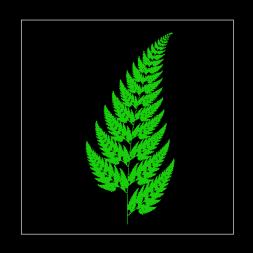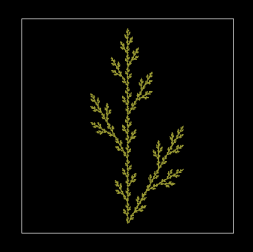a b c d e f p 0.14 0.01 0.00 0.51 -0.08 -1.31 0.10 0.43 0.52 -0.45 0.50 1.49 -0.75 0.35 0.45 -0.49 0.47 0.47 -1.62 -0.74 0.35 0.49 0.00 0.00 0.51 0.02 1.62 0.20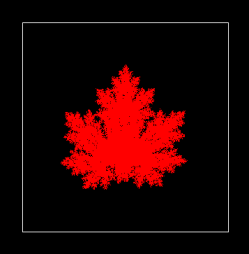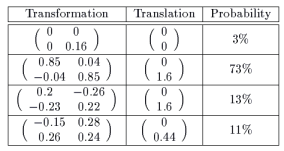The Collage Theorem
http://www.cut-the-knot.org/ctk/ifs.shtml

Fern Functions
http://mathworld.wolfram.com/BarnsleysFern.html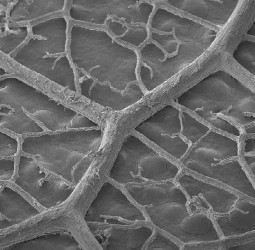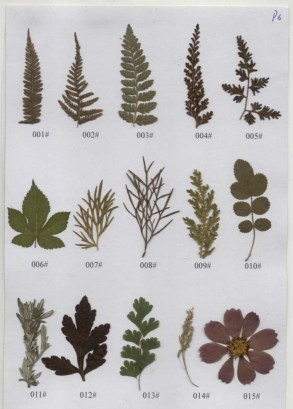http://fractalfoundation.org/OFC/OFC-12-1.html

http://fractalfoundation.org/OFC/OFC-12-2.html

http://fractalfoundation.org/OFC/OFC-12-3.html

http://sprott.physics.wisc.edu/carlson/

http://www.fractalus.com/gumbycat/

ICM 2006 Benoit Mandelbrot 碎形藝術競賽
http://www.fractalartcontests.com/2006/

Fractal Mathematics
http://www.hiddendimension.com/Mathematics_Main.html

Cynthia Lanius' Lessons: A Fractals Lesson - Introduction
http://math.rice.edu/~lanius/fractals/

http://wmi.math.u-szeged.hu/xaos/doku.php?id=main

Fractal Art
http://www.fractalus.com/info/manifesto.htm

http://math.rice.edu/~lanius/frac/

Fractals in Nature and How to Measure Them

Julia and Mandelbrot Sets (Clark University)
http://aleph0.clarku.edu/~djoyce/julia/index.html

http://en.wikipedia.org/wiki/Julia_set

http://en.wikipedia.org/wiki/De_Rham_curve

http://en.wikipedia.org/wiki/Iterated_function_system

BASIC 與自我類似 (Self-similarity)
http://www.geocities.jp/thinking_math_education/self-sim/self-sim.htm

True BASIC
http://www.truebasic.com/

Free BASIC Compilers and Interpreters
http://www.thefreecountry.com/compilers/basic.shtml